Solving Systems of Equations

 1. Solve the system:  y = -2x + 9   and   y = 3x - 4 1.  Enter the first equation into Y1. 2.  Enter the second equation into Y2. 3.  Hit GRAPH. 4.  Use the INTERSECT option to find where the two graphs intersect (the answer).      2nd TRACE (CALC) #5 intersect      Move spider close to the intersection.      Hit ENTER 3 times. 5.  Answer:  x = 2.6  and y = 3.8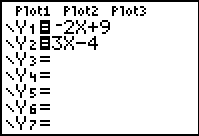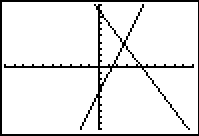2. Solve the system:  x - 2y = 14   and   x + 3y = 9 The graphing calculator will only accept entries that start with y = , so we need to solve these equations for y =.1.  Enter the first equation into Y1. 2.  Enter the second equation into Y2. 3.  Hit GRAPH.  The graphs appear to intersect OFF the window.  We need MORE x-values to the right hand side of the graph.  Go to WINDOW.  Increase the size of Xmax.  Hit GRAPH. 4.  Use the INTERSECT option to find where the two graphs intersect (the answer).      2nd TRACE (CALC) #5 intersect      Move spider close to the intersection.      Hit ENTER 3 times. 5.  Answer:  x = 12  and y = -1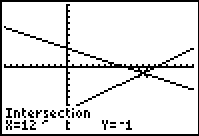Oops!!  They don't cross in the window.Better!  Xmax was increased to 20.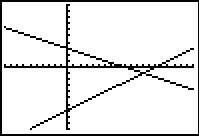3. Solve linear quadratic system:  y = x2 - 4x - 2  and   y = x - 2 1.  Enter the first equation into Y1. 2.  Enter the second equation into Y2. 3.  Hit GRAPH. 4.  Use the INTERSECT option twice to find the two locations where the graphs intersect (the answers).      2nd TRACE (CALC) #5 intersect      Move spider close to the intersection.      Hit ENTER 3 times. 5.  Answer:  (5,3)  and  (0,-2)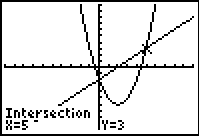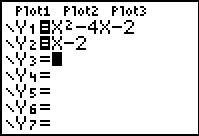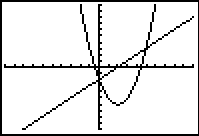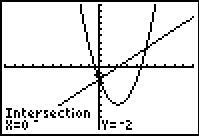Finding Your Way Around TABLE of  CONTENTS